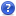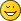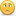General Algorithm for Primitive Calculation?

General Algorithm for Primitive Calculation?
#1Hi!

I just want to know if there exists an open source algorithm for the primitive generation...?

Okay... for rings and cones I have to round the sinus/cosinus to 4 decimal places (and then multiply it with a radius)...
...and round the result again to four dp (how should I round?)...

I know very well how to generate a (torus) primitive... but what are the precise numerical requirements for this task?
Is there any formal description which will lead to the same results on all machines?

What are the specified rounding modes?

Can I write a primitive generator program without a specification?

Currently, I am doing reverse engineering with PrimGen2...
...it seems to be (nearly) impossible to clone.

I make very good progress, but from time to time IEEE 754 pushes me back to the ground.
The mechanics of PrimGen2 are very clear to me and I know that .NET uses a different Math.Round() function than Java...

...it is not easy to finish the implementation soon.

Leg godt

Nils
RE: General Algorithm for Primitive Calculation?
#2
LDView is open source, and has primitive substitution for most LDraw primitives. Of course, it's in C++, and since it is using the values in-memory, it never rounds them, but it should be trivial to round to 4 decimal places (or whatever) during final output to the file. (You don't want to round anything except the final points written the file.)

The actual code in LDView is spread over a bunch of add... member functions in TREModel.cpp. Search for the following functions:
Note: numSegments would by 16 for typical LDraw; it's the total number of segments in a 360 degree circle; usedSegments is the number of segments to generate, so 4 for a quarter-circle when numSegments is 16.
RE: General Algorithm for Primitive Calculation?
#3
Quote:(You don't want to round anything except the final points written the file.)
That's not completely true. As Nils guessed, sin/cos of ring and cones primitives must be rounded before scaling them otherwise they wouldn't match other round primitives.

Quote:and round the result again to four dp (how should I round?)...
AFAIK it's simple rounding to nearest value. I beta tested Primgen2 and we struggled quite a bit to make it compatible with the original Primgen (for the sake of completeness Paul Easter Primgen is here http://www.geocities.ws/pneaster/prim-gen.html) while extending it and correcting its issues (mainly make the edge condlines tangent to plane/cylinder for cones and tori). I hope Mike will chime in but then... it's holidays seasonFYI: "Case study" of some numerical challenges (and the solution [not])
#4Thank you for your assistance! :)

I would like to be a little bit more technical with the following example.
Spoiler alert: I also got the solution while I was writing this text, so I possibly need no more help.
No, ... there is more work to do...

To be honest, I found a way to access the sourcecode from PrimGen2 without asking Mike for help ;)
Yeah... I was really curious how PrimGen2 works under hood...

So... lets get started with the ring primitives (I hope Mike will not hold this against me :D ):

Here is the hacked code from PrimGen2 (C#, CLR)
Code:
```for (int i = 0; i < Segments; i++) { objdat.LinePoint1.X = Math.Round(Math.Cos(((i * (360.0 / ((double) Divisions))) * 3.1415926535897931) / 180.0), 4) * InnerDiameter; objdat.LinePoint1.Y = 0.0; objdat.LinePoint1.Z = Math.Round(Math.Sin(((i * (360.0 / ((double) Divisions))) * 3.1415926535897931) / 180.0), 4) * InnerDiameter; objdat.LinePoint2.X = Math.Round(Math.Cos((((i + 1) * (360.0 / ((double) Divisions))) * 3.1415926535897931) / 180.0), 4) * InnerDiameter; objdat.LinePoint2.Y = 0.0; objdat.LinePoint2.Z = Math.Round(Math.Sin((((i + 1) * (360.0 / ((double) Divisions))) * 3.1415926535897931) / 180.0), 4) * InnerDiameter; objdat.LinePoint3.X = Math.Round(Math.Cos((((i + 1) * (360.0 / ((double) Divisions))) * 3.1415926535897931) / 180.0), 4) * (Width + InnerDiameter); objdat.LinePoint3.Y = 0.0; objdat.LinePoint3.Z = Math.Round(Math.Sin((((i + 1) * (360.0 / ((double) Divisions))) * 3.1415926535897931) / 180.0), 4) * (Width + InnerDiameter); objdat.LinePoint4.X = Math.Round(Math.Cos(((i * (360.0 / ((double) Divisions))) * 3.1415926535897931) / 180.0), 4) * (Width + InnerDiameter); objdat.LinePoint4.Y = 0.0; objdat.LinePoint4.Z = Math.Round(Math.Sin(((i * (360.0 / ((double) Divisions))) * 3.1415926535897931) / 180.0), 4) * (Width + InnerDiameter); // Generate output/objects here... }```

The function Math.Round(number, 4) rounds by default to even numbers (known as Banker's Rounding).
In this case, four digits are preserved.

Java uses another default rounding method. So I had to write .NET's Math.round() by myself. I called it round4f.

Code:
```private double round4f(double d) {    return new BigDecimal(d).setScale(4, RoundingMode.HALF_EVEN).doubleValue(); }```

There should be no precision loss (due to the use of the arbitrary BigDecimal class).

Well, as I coder, I might be a bit lazy sometimes... :)

I just copy & paste the C# code to Java and slightly modified it (I had to use my custom round function)...

And here is the code from LDPartEditor (Java, JVM)

Code:
```for (int i = 0; i < Segments; i++) { double objdatLinePoint1X = round4f(Math.cos(((i * (360.0 / ((double) Divisions))) * 3.1415926535897931) / 180.0)) * InnerDiameter; double objdatLinePoint1Y = 0.0; double objdatLinePoint1Z = round4f(Math.sin(((i * (360.0 / ((double) Divisions))) * 3.1415926535897931) / 180.0)) * InnerDiameter; double objdatLinePoint2X = round4f(Math.cos((((i + 1) * (360.0 / ((double) Divisions))) * 3.1415926535897931) / 180.0)) * InnerDiameter; double objdatLinePoint2Y = 0.0; double objdatLinePoint2Z = round4f(Math.sin((((i + 1) * (360.0 / ((double) Divisions))) * 3.1415926535897931) / 180.0)) * InnerDiameter; double objdatLinePoint3X = round4f(Math.cos((((i + 1) * (360.0 / ((double) Divisions))) * 3.1415926535897931) / 180.0)) * (Width + InnerDiameter); double objdatLinePoint3Y = 0.0; double objdatLinePoint3Z = round4f(Math.sin((((i + 1) * (360.0 / ((double) Divisions))) * 3.1415926535897931) / 180.0)) * (Width + InnerDiameter); double objdatLinePoint4X = round4f(Math.cos(((i * (360.0 / ((double) Divisions))) * 3.1415926535897931) / 180.0)) * (Width + InnerDiameter); double objdatLinePoint4Y = 0.0; double objdatLinePoint4Z = round4f(Math.sin(((i * (360.0 / ((double) Divisions))) * 3.1415926535897931) / 180.0)) * (Width + InnerDiameter); // Generate output/objects here... }```

However, someone must be very carful here, how to do the rounding for the output on the screen.

I build this off-wall ring with both tools.
The header is identical on LDPE and PrimGen2

Code:
```0 Ring  7.5 x 0.0056 Width 2.5 0 Name: 360\1-180ring7.5w2.5.dat 0 Author: Nils Schmidt [BlackBrick89] 0 !LDRAW_ORG Unofficial_Primitive 0 !LICENSE Redistributable under CCAL version 2.0 : see CAreadme.txt 0 BFC CERTIFY CCW```

...but when we take a look at the data from LDPE:

Code:
```4 16 10 0 0 9.998 0 0.175 7.4985 0 0.1313 7.5 0 0 4 16 9.998 0 0.175 9.994 0 0.349 7.4955 0 0.2617 7.4985 0 0.1313 0 // Build by LDPartEditor (PrimGen 2.X)```

...we can spot a 0.2617, and PrimGen outputs...

Code:
```4 16 10 0 0 9.998 0 0.175 7.4985 0 0.1313 7.5 0 0 4 16 9.998 0 0.175 9.994 0 0.349 7.4955 0 0.2618 7.4985 0 0.1313 0 // Build by Primitive Generator 2```

...suddenly 0.2618 appears! This technically not equal to 0.2617. With some effort, you'll see that 0.2617 is in reality a 0.26175. It should be rounded to 0.2618...

First, I used the DecimalFormat class to archive the classical "half up" rounding with an output of four decimal places.

...strangely, it did not round with "half up"... even after setting the rounding mode accordingly...
so I had to write my own interpretation of a DecimalFormatter...

and now it works :)

[size=large]It doesn't.[/size]

I got a 0.5036499999999999.

LDPE says it's 0.5036.
PrimGen2 says it's 0.5037.

Arrgh!
RE: FYI: "Case study" of some numerical challenges (and the solution [not])
#5
Quote:I got a 0.5036499999999999.

LDPE says it's 0.5036.
PrimGen2 says it's 0.5037.

Arrgh!
I feel your pain... At least I learnt things about roundingLooks like we were lucky when we cloned the original primgen, once we figured out things, file comparison sturned out OK. Or maybe we didin't stumble on a tricky case? Now I am not sure that a 1/10000 error from time to time would be a real issue...
This last problem is related to this https://en.wikipedia.org/wiki/Rounding#T...7s_dilemma ?
I had the idea of rounding twice...
#6
(2016-08-12, 5:46)Philippe Hurbain Wrote:
Quote:I got a 0.5036499999999999.

LDPE says it's 0.5036.
PrimGen2 says it's 0.5037.

Arrgh!
I feel your pain... At least I learnt things about rounding ;)
Looks like we were lucky when we cloned the original primgen, once we figured out things, file comparison sturned out OK. Or maybe we didin't stumble on a tricky case? Now I am not sure that a 1/10000 error from time to time would be a real issue...
This last problem is related to this https://en.wikipedia.org/wiki/Rounding#T...7s_dilemma ?

Finally, I found a practical solution to this :)
I simply round twice.

Code:
```private String formatDec(double d) { // d is 0.00004999999 (string representation)  return bigDecimalToString(         new BigDecimal(String.valueOf(d))         .setScale(8, RoundingMode.HALF_UP)      // Round to 8 decimal places (to transform 0.00004999999 into 0.00005)         .setScale(4, RoundingMode.HALF_UP));    // Round to 4 decimal places (0.00005 -> 0.0001) }```

Assume that N is < 9 and X > 4.
This will convert any 0.0000N999X number to the next 0.0000(N+1) number. It solves the problem for the trailing 9's.
Okay.. 0.000049990 will not work, but I guess this has no practical impact.

Just for demonstration, the following primitve is now identical in both tools (PrimGen2 and LDPE):

Code:
```0 Torus Outside  1 x 0.5455 x 1 0 Name: 17\t01o5455.dat 0 Author: Primitive Generator 2 0 !LDRAW_ORG Unofficial_Primitive 0 !LICENSE Redistributable under CCAL version 2.0 : see CAreadme.txt 0 BFC CERTIFY CCW 0 // Major Radius: 11 0 // Tube(Minor) Radius: 6 0 // Segments(Sweep): 17/17 = 1.00 0 // 1  9  0 0 0  1 0 0  0 1 0  0 0 1 4-4edge.dat 0 // 1  12  1 0 0 0.5455 0 0  0 0 0.5455  0 1 0  4-4edge.dat```

Nobody would even need that torus...
Nevertheless, I can sleep well again ;)
RE: I had the idea of rounding twice...
#7
I'm not positive, but the "correct" solution might be your original round4f, with one minor change. Instead of constructing a BigDecimal with just the double value (for arbitrary precision), construct it with the double value and a MathContext.DECIMAL64 as a second argument. Based on the documentation, this should make BigDecimal use IEEE 754 rounding rules, which I would expect to match the C# rounding.

Side note: I'm pretty sure your solution can trigger wrong rounding in other cases. Say, for example, that your calculated value is 0.5036499999999911 instead of 0.5036499999999999. You're going to round up to 0.5037 in that case, while 0.5036 would be the correct result. Also, it seems that you could avoid the whole string thing by rounding the BigDecimal to 8 places, and then rounding that rounded BigDecimal to 4 places.
RE: I had the idea of rounding twice...
#8
(2016-08-12, 19:48)Travis Cobbs Wrote: I'm not positive, but the "correct" solution might be your original round4f, with one minor change. Instead of constructing a BigDecimal with just the double value (for arbitrary precision), construct it with the double value and a MathContext.DECIMAL64 as a second argument. Based on the documentation, this should make BigDecimal use IEEE 754 rounding rules, which I would expect to match the C# rounding.

Side note: I'm pretty sure your solution can trigger wrong rounding in other cases. Say, for example, that your calculated value is 0.5036499999999911 instead of 0.5036499999999999. You're going to round up to 0.5037 in that case, while 0.5036 would be the correct result. Also, it seems that you could avoid the whole string thing by rounding the BigDecimal to 8 places, and then rounding that rounded BigDecimal to 4 places.

Thanks!
I tried a few things and finally released the PrimGen2 clone with LDPartEditor 0.8.22.
At the beginning, I thought it would be quite simple to generate some LDraw primitives...
...it turns out to be another lecture in numerics instead :)
« Next Oldest | Next Newest »

Forum Jump:

Users browsing this thread: 1 Guest(s)